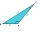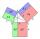Greatest angle

Calculate the greatest triangle angle with sides 197, 208, 299.

Result

α =  95.1 °

Solution:Try calculation via our triangle calculator.

Leave us a comment of example and its solution (i.e. if it is still somewhat unclear...):Be the first to comment!To solve this example are needed these knowledge from mathematics:

Cosine rule uses trigonometric SAS triangle calculator. See also our trigonometric triangle calculator.

Next similar examples:

1. Four sides of trapezoidIn the trapezoid ABCD is |AB| = 73.6 mm; |BC| = 57 mm; |CD| = 60 mm; |AD| = 58.6 mm. Calculate the size of its interior angles.
2. Side cIn △ABC a=2, b=4 and ∠C=100°. Calculate length of the side c.
3. Calculate 2Calculate the largest angle of the triangle whose side are 5.2cm, 3.6cm, and 2.1cm
4. Angles by cosine lawCalculate the size of the angles of the triangle ABC, if it is given by: a = 3 cm; b = 5 cm; c = 7 cm (use the sine and cosine theorem).
5. DiagonalsCalculate the length of the diagonals of the rhombus if its side is long 5 and one of its internal angle is 80°.
6. Diagonals in diamondIn the rhombus is given a = 160 cm, alpha = 60 degrees. Calculate the length of the diagonals.
7. Clock faceclock face is given. Numbers 10 and 5, and 3 and 8 are connected by straight lines. Calculate the size of their angles.
8. Scalar dot productCalculate u.v if |u| = 5, |v| = 2 and when angle between the vectors u, v is: a) 60° b) 45° c) 120°
9. Scalene triangleSolve the triangle: A = 50°, b = 13, c = 6
10. Vector sumThe magnitude of the vector u is 12 and the magnitude of the vector v is 8. Angle between vectors is 61°. What is the magnitude of the vector u + v?
11. The pondWe can see the pond at an angle 65°37'. Its end points are 155 m and 177 m away from the observer. What is the width of the pond?
12. FlowerbedFlowerbed has the shape of an isosceles obtuse triangle. Arm has a size 5.5 meters and an angle opposite to the base size is 94°. What is the distance from the base to opposite vertex?
13. AnglesIn the triangle ABC, the ratio of angles is: a:b = 4: 5. The angle c is 36°. How big are the angles a, b?
14. In a 2In a thirteen sided polygon, the sum of five angles is 1274°, four of the eight angles remaining are equal and the other four are 18° less than each of the equal angles. Find the angles. .
15. Reference angleFind the reference angle of each angle:
16. LawsFrom which law follows directly the validity of Pythagoras' theorem in the right triangle? ?
17. Theorem proveWe want to prove the sentence: If the natural number n is divisible by six, then n is divisible by three. From what assumption we started?